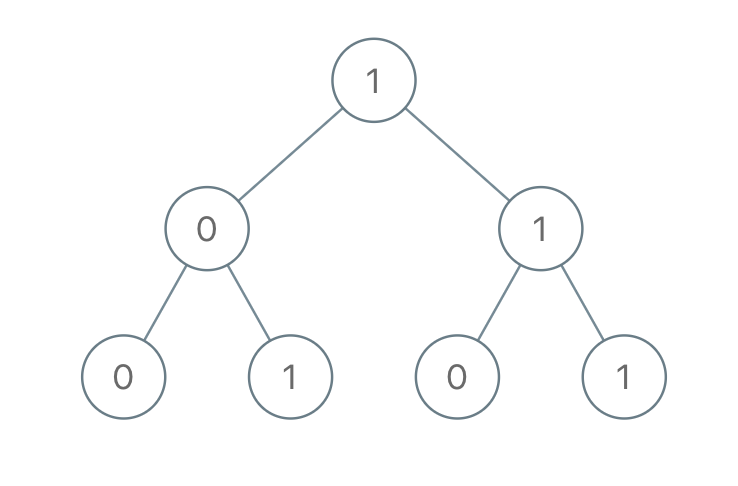1022. Sum of Root To Leaf Binary Numbers

You are given the `root` of a binary tree where each node has a value `0` or `1`.  Each root-to-leaf path represents a binary number starting with the most significant bit.  For example, if the path is `0 -> 1 -> 1 -> 0 -> 1`, then this could represent `01101` in binary, which is `13`.

For all leaves in the tree, consider the numbers represented by the path from the root to that leaf.

Return the sum of these numbers. The answer is guaranteed to fit in a 32-bits integer.

Example 1:```Input: root = [1,0,1,0,1,0,1]
Output: 22
Explanation: (100) + (101) + (110) + (111) = 4 + 5 + 6 + 7 = 22
```

Example 2:

```Input: root = 
Output: 0
```

Example 3:

```Input: root = 
Output: 1
```

Example 4:

```Input: root = [1,1]
Output: 3
```

Constraints:

• The number of nodes in the tree is in the range `[1, 1000]`.
• `Node.val` is `0` or `1`.

1022. Sum of Root To Leaf Binary Numbers
``````struct Solution;
use rustgym_util::*;

impl Solution {
fn sum_root_to_leaf(root: TreeLink) -> i32 {
let mut sum = 0;
Self::sum_r(&root, 0, &mut sum);
sum
}
if let Some(node) = link {
let node = node.borrow();
let left = &node.left;
let right = &node.right;
let val = node.val + parent_val * 2;
if left.is_none() && right.is_none() {
*sum += val;
} else {
Self::sum_r(left, val, sum);
Self::sum_r(right, val, sum);
}
}
}
}

#[test]
fn test() {
let root = tree!(
1,
tree!(0, tree!(0), tree!(1)),
tree!(1, tree!(0), tree!(1))
);
assert_eq!(Solution::sum_root_to_leaf(root), 22);
}
``````